Courses

# Test: Communication Systems - 7

## 25 Questions MCQ Test Communication Theory | Test: Communication Systems - 7

Description
This mock test of Test: Communication Systems - 7 for Electronics and Communication Engineering (ECE) helps you for every Electronics and Communication Engineering (ECE) entrance exam. This contains 25 Multiple Choice Questions for Electronics and Communication Engineering (ECE) Test: Communication Systems - 7 (mcq) to study with solutions a complete question bank. The solved questions answers in this Test: Communication Systems - 7 quiz give you a good mix of easy questions and tough questions. Electronics and Communication Engineering (ECE) students definitely take this Test: Communication Systems - 7 exercise for a better result in the exam. You can find other Test: Communication Systems - 7 extra questions, long questions & short questions for Electronics and Communication Engineering (ECE) on EduRev as well by searching above.
QUESTION: 1

### Consider the following test signal patterns Ramp Grill Grey shades Sine pulse Picture line up generating equipment Which of the above are used in TV studios?

Solution:

All these are standard test signals used in TV studios.

QUESTION: 2

### If aspect ratio is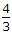and number of lines in each frame is 625, the number of picture elements in one frame is

Solution:

Since there are 625 lines in one frame, the number of picture elements is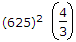.

QUESTION: 3

### The resonant frequency of an RF amplifier is 1 MHz and its bandwidth is 10 kHz. The Q factor will be

Solution: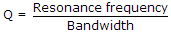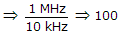.

QUESTION: 4

Indicate the false statement: If SWR on a transmission line is infinite, the line is terminated in

Solution:

If line is OC or SC or terminated in pure reactance, SWR is 1.

QUESTION: 5

In TV signals colour burst is used

Solution:

Colour burst consists of a short train of about 10 cycles sent to the receiver along with synchronous signals. It ensures I and Q phase correctly.

QUESTION: 6

The total number of pulses in a TV remote control is

Solution:

The 14 pulses are preliminary pulse, start pulse, stop pulse and 11 other pulses.

QUESTION: 7

Assertion (A): A Smith chart can be used for a number of calculations of a transmission line

Reason (R): Smith chart is basically a polar impedance diagram.

Solution:

Smith chart is a convenient tool for transmission line design and analysis.

QUESTION: 8

Which camera tube has highest sensitivity and very wide spectral response?

Solution:

New vicon has very high sensitivity and very wide spectral response which extends well into infrared region.

QUESTION: 9

Consider the following functions

1. It detects the AM video carrier IF so as to produce the video signal
2. It produces and separates out the inter carrier sound IF of 5.5 MHz
3. It amplifies the video signal
4. It amplifies the audio signal

The video detector in a colour TV performs which of the above functions

Solution:

Video detector does not amplify any signal.

QUESTION: 10

A computer monitor uses 180 W in normal operation and 20 W in sleep mode. The computer is on all the 24 hours but is in sleep mode for 18 hours a days. The energy units saved in 30 days are

Solution: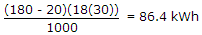.

QUESTION: 11

Which of the following antennas is circularly polarized?

Solution:

The helical antenna is circularly polarized, i.e., its polarization is evenly divided between vertical and horizontal components.

QUESTION: 12

In colour TV system the colour sub carrier frequency is 4.43 MHz.

Solution:

Video monitor does not have RF tuner and IF amplifier stages.

QUESTION: 13

A transmitter power of 5 W is increased by 20 dB. The effective radiated power is

Solution: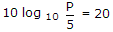or P = 500 W.

QUESTION: 14

The percentage saving in power of 100% modulated suppressed carrier AM signal is

Solution:

m = 1,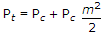if carrier is suppressed then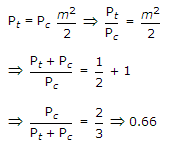.

QUESTION: 15

The maximum distance between television transmission and receiving antennas for reasonably good reception should be

Solution:

Television transmission is governed by line of sight distance which is about 100 km or less.

QUESTION: 16

Assertion (A): EM waves are attenuated as they travel outward from a source

Reason (R): Both field intensity and power density follow inverse square law.

Solution:

As per inverse square law, power is inversely proportional to square of distance.

QUESTION: 17

The frequency response of a microphone means

Solution:

100 Hz is taken as standard for specifying the output.

QUESTION: 18

Graphic equaliser system in stereophonic systems generally have

Solution:

Stereo systems have 3 or 4 bands at 300 Hz, 1000 Hz, 10000 Hz etc. in graphic equaliser system.

QUESTION: 19

An amplifier operating over a frequency range of 18 to 20 MHz has 10 kΩ input resistance. At a temperature of 27º C, the noise voltage at input of amplifier is (Boltzman constant k = 1.38 x 10-23 J/K)

Solution:

Using Equation Va = (4 x 1.38 x 10-23 x 300 x 2 x 106 x 104)0.5 = 18.2 x 10-6 V.

QUESTION: 20

Some sources of TV programs are

1. TV camera
2. Telecine
3. VTR
4. External signal

Which of the above are actually present in a TV studio?

Solution:

All these are present.

QUESTION: 21

Two sinusoidal signals of same amplitude and frequencies 10 kHz and 10.1 kHz are added together. The combined signal is given to an ideal frequency detector. The output of the detector is

Solution:

Y(t) = A cos (2p x 104 t) + A cos (2p x 10.1 x 103 t)

when passed through high pass filter,

Output will be A cos (2p x 10.1 x 103 t) only.

QUESTION: 22

In Vidicon camera tube, the resistance of photoconductive material on the target plate

Solution:

Light dependent resistor (LDR) has high resistance in darkness and low resistance in brightness.

QUESTION: 23

In a satellite transponder the power amplifier feeds power P to the transmitting antenna of gain G. If distance of earth is d, the power flux density is

Solution:

Available power is PG and is spread over 4 nd2.

QUESTION: 24

The percentage saving in power of 100% modulated suppressed carrier AM signal is

Solution:

Pc = 400 W, m = 0.75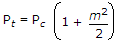QUESTION: 25

Which of the following are useful in comparing the noise performance of receivers?

1. Input noise voltage
2. Noise figure
3. Noise resistance
4. Noise temperature

Select answer as per the following codes:

Solution:

Noise figure, noise resistance and noise temperature are useful quantities. Input noise voltage alone is not sufficient unless gain of amplifier stages is also known.